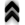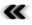### Organization

A fundamental part of scientiﬁc computing is related to the transformation of physical ﬁeld problems into discrete mathematical problems, which can then be solved on digital computers. This transition is mirrored in a two part organization of this work.

Before proceeding in elaborations about scientiﬁc computing, a short outline, what the term should be understood as, is provided in order to reinforce foundations upon which the remainder is built. Scientiﬁc computing aspires to complement the classical methods of scientiﬁc investigations, namely theory and experiment. It can accomplish this by applying the facilities made available by digital computers to the ﬁelds of scientiﬁc theories. Since this procedure can be applied to virtually every science whose theory can be modelled using mathematical methods the requirements are very disparate due to the vast ﬁeld of problem domains. Thus, it is imperative to have ﬂexibility and reusability in mind, when dealing with the ﬁeld of scientiﬁc computing, in order to prevent a fracture of the relatively young ﬁeld into several distinct disciplines. This issue is further complicated as scientiﬁc computing is applied not to the easiest of tasks, but focuses on problems which result in vast amounts of data and high computational needs, thus eﬃciency in terms of computational resources can obviously not be neglected. Flexibility is again called for in order to make adaptations to changes in available resources as easy as possible.Figure 1.1: Organization of chapters and parts.

The thesis is subdiveded into 8 Chapters. The general structuring of chapters is illustrated in Figure 1.1. Chapter 2 establishes the value of abstraction necessary for eﬃcient scientiﬁc computing and prepares for the contributions and results of this work. Chapter 3 gives an outline of the options available for implementations. It furthermore motivates the choice of programming language used throughout the remainder of the thesis. The chapter concludes giving an illustrative example application using generic programming.

The main bulk of this document is split into two dual parts. While the two parts are distinct, they are linked to one another by being designed to mirror each other regarding topics and concepts of the contained Chapters.

Part II contains Chapter 4 and Chapter 5 which provide theoretical background for further exploits. In particular Chapter 4 introduces terms and deﬁnitions, and the overall mathematical apparatus, which forms the intellectual backbone. It is the result of a reﬁnement of information acquired in a hunter-gather style from various sources to produce a self contained and consistent compilation, which had not been available in the context of scientiﬁc computing. Where Chapter 4 deals with the bare mathematical structures, even when already hinting at applicability, Chapter 5 concerns itself with the establishment of models from a physical perspective. It already draws on the mathematical formalisms previously made available and is thus relatively concise. Section 5.7 may be of special interest, as it represents a departure from the standard introduction of quantum mechanics in order to better illustrate similarities between the classical and the quantum case.

In contrast to these theoretical aspects, Part III deals with methodologies of utilizing them in order to create the abstract realizations of implementations. Chapter 2 not only reiterates basic motivating ideas, but also describes caveats when dealing with abstractions. The chapter ﬁnally presents the idea of using generic programming guided by mathematical concepts to organize implementations for scientiﬁc computing.

Since the chapters of Part III are able to draw on a ﬁrm foundation of terms and deﬁnitions from Part II, they can be kept conveniently concise, thus avoiding unnecessary distractions. For ease of association Chapter 6 and Chapter 7 follow the outline presented in the chapters of Part II, as indicated in Figure 1.1. Thus, Chapter 6 shows examples of realizing the mathematical concepts of Chapter 4 mainly using the generic programming paradigm in the C++ language. Where Chapter 4 is the backbone of the thesis as a whole, Chapter 6 is the structuring backbone of the implementation examples of Chapter 7, which reﬂect implications from the theoretical Chapter 5. As such, where the examples of Chapter 6 represent instances of mathematical concepts to form components, Chapter 7 assembles components and applies them to the problem domain of physics.

At ﬁrst the focus is on examples illustrating how basic components are combined to form applicable modules for physically motivated sub-problems before applying basic components to examples providing illustrative results. The ﬁnal sample application combines not only the previously introduced software components, but also is physically challenging, where the other examples have been kept simple not to detract from implementation issues. Chapter 8 concludes with attempting to turn to the gloom yet to come.

Having established the basic ﬁeld in Chapter 3, the executing part on computing machines and the rising of abstraction by algorithms and mathematics, Part II not only introduces the necessary foundation for strict and formal mathematical structures to formalize the modelling of algorithms, but also consolidates diﬀerent areas of mathematics. Own contributions are reﬂected by reﬁnement and convergence of available structures and methods, to provide compatible, realizable, and eﬀective abstraction levels in Part III, which translates the consolidated concepts from Part II to the area of discrete and ﬁnite resources present in digital computers.

Chapter 6 initiates the translation of mathematical structures into applicable components and contributions to a software environment. Several data structures are presented to model topological spaces and ﬁber bundles as well as the application of geometrical properties. Special consideration is placed on alternate methods for integration in Section 6.6. Applications of Chapter 5 are outlined in Chapter 7, which also presents additional contributions of this work. In particular, Section 7.1 discusses dynamical systems due to Boltzmann’s equation in a setting of diﬀerential equations and an important area of solid state physics, semiconductor analysis, is further analysed regarding macroscopic descriptions of electrons.

In contrast to the local setting of Section 7.1, Section 7.2 derives a direct approach to use the phase space and its geometry applying the integral form of Boltzmann’s equation as introduced in Section 5.6. Additional contributions are then presented by casting sections of trajectories through the phase space and enabling trajectory transitions. Application examples are demonstrated by using modern programming techniques to not only enable the integration of already existing and well-tested code, but also allowing the seamless transition from already developed applications to new applications, thus enabling the use of modern hardware features such as parallelization and GPU computing.

Where previous parts of this work regarding implementations deal with classical settings, Section 7.3 alters the discussion to non-local coherent phenomena, contributing an alternate, scattering induced, correction to quantum mechanics (Section 5.7). Finally a resonant tunnelling diode example is presented in Section 7.4 to show approximations to quantum eﬀects in semiconductor devices.